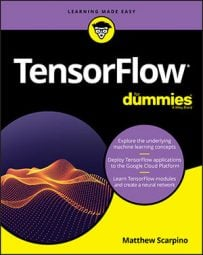##### TensorFlow For DummiesAn application must specify the shape of each tensor to be created. The `tf` package provides functions that update tensors and their shapes after creation. This table lists these transformation functions and provides a description of each.

Functions for Transforming Tensors

 Function Description `cast(tensor, dtype, name=None)` Changes the tensor's data type to the given type `reshape(tensor, shape, name=None)` Returns a tensor with the same elements as the given tensor with the given shape `squeeze(tensor, axis=None, name=None, squeeze_dims=None)` Removes dimensions of size 1 `reverse(tensor, axis, name=None)` Reverses given dimensions of the tensor `slice(tensor, begin, size, name=None)` Extracts a portion of a tensor `stack(tensors, axis=0, name='stack')` Combines a list of tensors into a tensor of greater rank `unstack(tensor, num=None, axis=0, name='unstack')` Splits a tensor into a list of tensors of lesser rank
Despite its name, reshape doesn't modify an existing tensor. Instead, the function returns a tensor with the same elements as the given tensor and the specified shape. For example, the following code uses `reshape `to convert a four-element vector into a 2-x-2 matrix:
`vec = tf.constant([1., 2., 3., 4.])mat = tf.reshape(vec, [2, 2])<strong># Result: [[1. 2.], [3. 4.]]</strong>`
If any dimension of a tensor has a size of 1, calling `squeeze `will remove it from the tensor, thereby reducing the tensor's rank. If the function’s `axis `parameter identifies one or more dimensions, only those dimensions will be affected by `squeeze`.

In the `reverse `function, the `axis `parameter identifies one or more dimensions to be reversed. The following code demonstrates how `reverse `works:

`mat = tf.constant([[1., 2., 3.], [4., 5., 6.]])rev_mat = tf.reverse(end, )<strong># Result: [[4. 5. 6.], [1. 2. 3.]]</strong> rev_mat = tf.reverse(end, )<strong># Result: [[3. 2. 1.], [6. 5. 4.]]</strong> rev_mat = tf.reverse(end, [0, 1])<strong># Result: [[6. 5. 4.], [3. 2. 1.]]</strong>`
The `slice `function extracts subtensors from a tensor. The `begin `parameter identifies the index of the first element to be extracted, and `size `identifies the shape of the tensor to be extracted, starting from the `begin `location.

For example, suppose that you want to extract the lower-right 2-x-2 matrix from a 3-x-3 matrix. The index of the first extracted element is [1, 1] and the size of the desired tensor is [2, 2]. The following code uses `slice `to perform this extraction:

`mat =tf.constant([[1., 2., 3.], [4., 5., 6.], [7., 8., 9.]])slice_mat = tf.slice(mat, [1, 1], [2, 2])<strong># Result: [[5. 6.] [7. 8.]]</strong>`
`stack `accepts a list of tensors of rank N and returns a single tensor of rank N+1. In addition to having the same rank, the input tensors must have the same shape. The following code demonstrates how `stack `can be used. The function combines three one-dimensional tensors into a two-dimensional tensor:
`t1 = tf.constant([1., 2.])t2 = tf.constant([3., 4.])t3 = tf.constant([5., 6.])t4 = tf.stack([t1, t2, t3])`
When these operations execute, `t4` will equal [[1. 2.] [3. 4.] [5. 6.]]. If the axis parameter is set to 1, stacking will be performed along the second dimension, so t4 will set to [[1. 3. 5.] [2. 4. 6.]].

`unstack `performs the inverse operation of `stack`. That is, unstack accepts a tensor of rank N and returns a list of tensors of rank N-1. The num parameter determines how many tensors should be unpacked, and if this isn't set, `unstack `infers the number from the tensor’s shape.